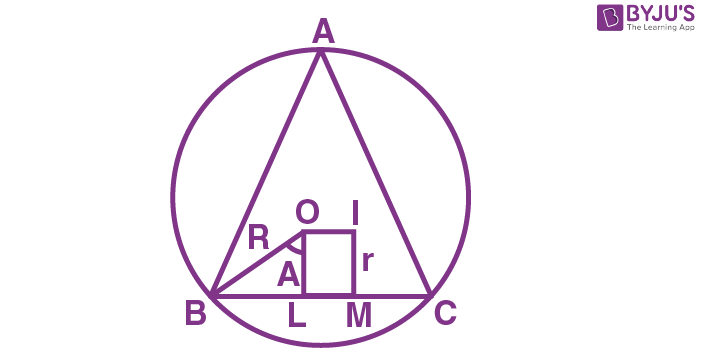Checkout JEE MAINS 2022 Question Paper Analysis : Checkout JEE MAINS 2022 Question Paper Analysis :

# If in a triangle ABC, the line joining the circumcentre O and the incentre I is parallel to BC, then

1) r = R cos A

2) r = R sin A

3) R = r cos A

4) R = r sin A

Solution:Let I denote the incentre and O denotes the circumcentre.

OI is parallel to BC

O and I are equidistant from BC

OL = IM = r (OL ⊥ BC, IM ⊥ BC)

∠BOL = ½ ∠BOC = A

OB = R

From ∆OBL, cos A = OL/OB

= OL/R

OL = R cos A

IM = R cos A

So r = R cos A

Hence option (1) is the answer.(1)(0)